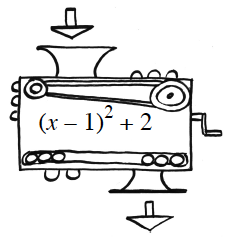### Home > INT2 > Chapter 9 > Lesson 9.3.3 > Problem9-108

9-108.The number $6$ is the output for Carmen’s function machine shown at right. What number(s) could have been dropped in?

Since $6$ is the output, set the expression inside of the function machine equal to $6$.

$(x - 1)^2 + 2 = 6$

$x^2 - 2x = 3$

$x(x - 2) = 3$

Use the Zero Product Property: $x = -1$, $x = 3$